# Introduction to Matplotlib and Seaborn

## Introduction

In this blog, I'll cover the basics of matplotlib and seaborn, such as what they are, why they're used, how to get started with them, different operations they can perform, and a comparison of the two.

## Matplotlib

Matplotlib is a fantastic Python visualization package for 2D array plots. Matplotlib is a NumPy-based multi-platform data visualization framework. Matplotlib has a variety of plots such as line, bar, scatter, histogram, etc.

## Getting started with Matplotlib

### Installation

``pip install matplotlib``

### Importing Matplotlib

``````from matplotlib import pyplot as plt
""" or """
import matplotlib.pyplot as plt``````

### Common plots in Matplotlib

``````# values of x-axis
x = [7, 3, 9, 5, 8]
# values of y-axis
y = [8, 4, 6, 3, 1]``````

#### Line plot

``````# Plotting Function
plt.plot(x, y)

# display the plot
plt.show()``````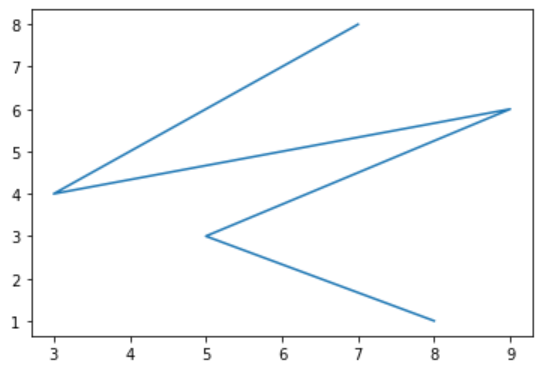#### Scatter plot

``````# Plotting Function
plt.scatter(x, y)

# display the plot
plt.show()``````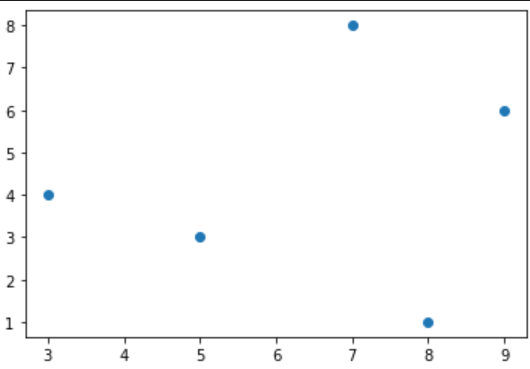#### Histogram plot

``````# Plotting Function
plt.hist(x)

# display the plot
plt.show()``````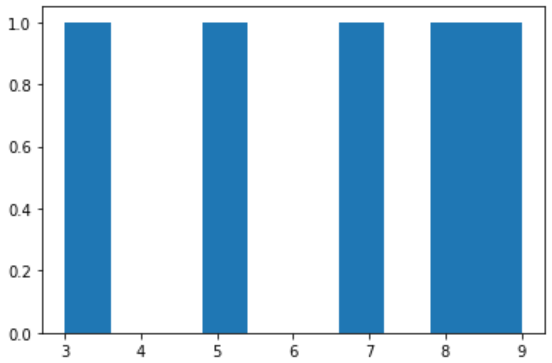#### Bar plot

``````# Plotting Function
plt.bar(x, y)

# display the plot
plt.show()``````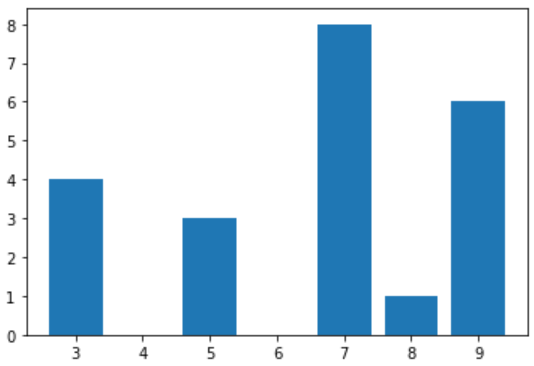## Seaborn

Seaborn is an excellent Python visualization tool for plotting statistical visuals. It is based on the matplotlib software and is tightly connected with pandas data structures.

The goal of Seaborn is to make visualization a core aspect of data exploration and understanding. It offers dataset-oriented APIs, allowing us to move between several visual representations for the same variables to comprehend the data better.

## Getting started with Seaborn

### Installation

``pip install Seaborn``

### Importing Seaborn

``````import numpy as np

import seaborn as sns``````

### Common plots in Seaborn

#### Line plot

``````sns.set(style='dark')

# getting the dataset

# Plotting for different events regions
sns.lineplot(x='timepoint', y='signal', hue='region', style='event', data=fmri)``````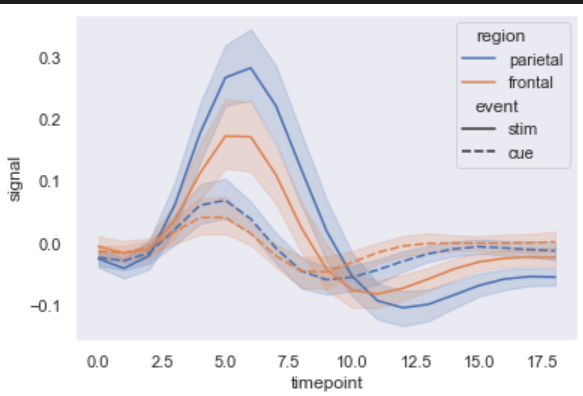#### Lmplot plot

``````sns.set(style='dark')

# getting the dataset

# plotting the results
sns.lmplot(x='x', y='y', data=data)``````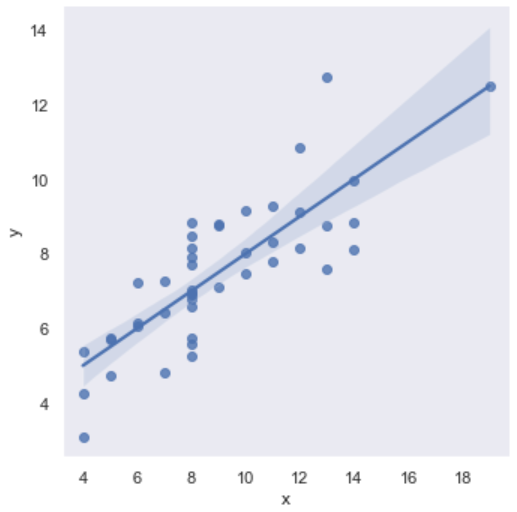#### Dist plot

``````# chosing style as white (other options - dark, whitegrid, darkgrid and ticks)
sns.set(style='white')

# generate a random dataset
data = np.random.RandomState(10)
ndata = data.normal(size=100)

# Plotting a histogram
sns.distplot(ndata, kde=True, color='m')``````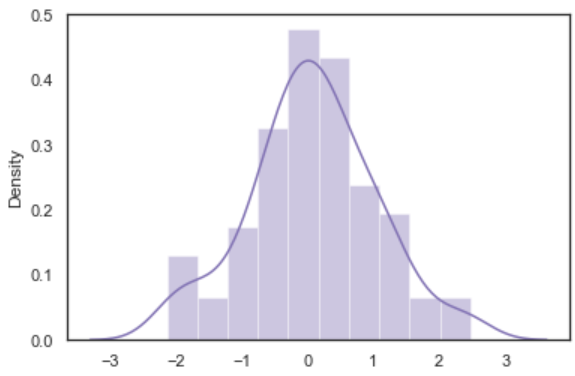## Seaborn vs Matplotlib

• Functionality
• Seaborn: Seaborn has many visualization patterns to choose from. It employs less syntax and comes with several visually appealing default themes. It specializes in statistics visualization and is used when a user needs to summarise data in a visualization while simultaneously displaying the data's distribution.
• Matplotlib: Matplotlib is primarily used for simple plotting. Bars, pies, lines, scatter plots, and other visualizations using Matplotlib are standard.
• Managing multiple figures
• Seaborn: The generation of several figures is automated by Seaborn. It can cause OOM (out of memory) problems.
• Matplotlib: In matplotlib, we can open multiple figures, but every figure must be closed explicitly.
• Data frames and Arrays
• Seaborn: Seaborn is considerably more intuitive than Matplotlib because it works with the entire dataset. Replot() is the entry API for Seaborn, with the 'kind' parameter allowing you to define the type of plot, which can be line, bar, or any of the other options. Seaborn is not stateful. Therefore, passing an object is required in plot().
• Matplotlib: Matplotlib works with arrays and data frames. For plotting, it has a variety of stateful APIs. Because the object represents the figures and aces, plot() calls without parameters work, eliminating the need to manage parameters.
• Flexibility
• Seaborn: Seaborn reduces repetition by providing commonly used default themes.
• Matplotlib: Matplotlib is a flexible and powerful plotting library.

## FAQs

1. What are the different categories of plot in Seaborn?
Seaborn has several categories of plots, such as Relational Plots, Categorical Plots, Regression Plots, etc.

2. How to open and display images using matplotlib?
``````# importing required libraries
import matplotlib.pyplot as plt
import matplotlib.image as img

# displaying the image
plt.imshow(testImage)``````

## Key Takeaways

Cheers if you reached here!! In this blog, we used a random dataset to understand how the K-Means clustering algorithm works.

Yet learning never stops, and there is a lot more to learn. Happy Learning!!